# Identity Mappings in Deep Residual Networks（译）

## 介绍

yl=h(xl)+F(xl,Wl),xl+1=f(yl),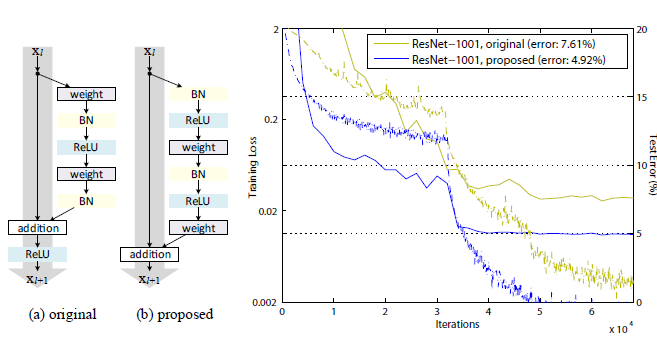Fig.1 (a) 原始残差单元；(b) 本文提出的残差单元；右：1001层ResNets 在CIFAR-10上的训练曲线。实线对应测试误差(右侧的y轴)，虚线对应训练损失(左侧的y轴)。本文提出的单元使得ResNet-1001的训练更简单。

## Analysis of Deep Residual Networks

He2016中的ResNets是通过将相同连接形状的blocks进行堆叠的模块化框架。本文中，我们将这些blocks称为“残差单元(Residual Units)”。He2016中的原始残差单元执行以下计算(Eq.1，Eq.2)：

yl=h(xl)+F(xl,Wl),xl+1=f(yl).

xl+1=xl+F(xl,Wl).

xL=xl+i=lL1F(xi,Wi),

Eq.4展现了一些良好的特性。(i) 对于任意深的单元L$L$的特征 xL${x}_L$ 可以表达为浅层单元l$l$ 的特征 xl${x}_l$加上一个形如 L1i=lF$\sum_{i=l}^{L-1}\mathcal{F}$的残差函数，这表明了任意单元L$L$l$l$之间都具有残差特性。(ii) 对于任意深的单元L$L$，它的特征 xL=x0+L1i=0F(xi,Wi)${x}_{L} = {x}_{0} + \sum_{i=0}^{L-1}\mathcal{F}({x}_{i}, \mathcal{W}_{i})$，即为之前所有残差函数输出的总和(加上x0${x}_{0}$)。而正好相反的是，“plain network”中的特征xL${x}_{L}$是一系列矩阵向量的乘积，也就是L1i=0Wix0$\prod_{i=0}^{L-1}W_{i}{x}_0$ (忽略了BN和ReLU)。

Eq.4同样也具有良好的反向传播特性。

Exl=ExLxLxl=ExL(1+L1i=lF(xi,Wi)xl).

Eq.5表明了梯度Exl$\frac{\partial E}{\partial {{x}_{l}}}$可以被分解成两个部分：其中ExL$\frac{\partial E}{\partial {{x}_{L}}}$直接传递信息而不涉及任何权重层，而另一部分ExL(L1i=lFxl)$\frac{\partial E}{\partial {{x}_{L}}}\left(\frac{\partial {\sum_{i=l}^{L-1}\mathcal{F}}}{\partial {{x}_{l}}}\right)$表示通过权重层的传递。ExL$\frac{\partial E}{\partial {{x}_{L}}}$保证了信息能够直接传回任意浅层 l$l$
Eq.5同样表明了在一个mini-batch中梯度Exl$\frac{\partial E}{\partial {{x}_{l}}}$不可能出现消失的情况，因为通常L1i=lFxl$\frac{\partial {\sum_{i=l}^{L-1}\mathcal{F}}}{\partial {{x}_{l}}}$对于一个mini-batch总的全部样本不可能都为-1。这意味着，哪怕权重是任意小的，也不可能出现梯度消失的情况。

Discussions
Eq.4和 Eq.5表明了，在前向和反向阶段，信号都能够直接的从一个单元传递到其他任意一个单元。Eq.4的条件基础是两个恒等映射： (i) 恒等跳跃连接h(xl)=xl$h({x}_{l}) = {x}_{l}$，和(ii) f$f$ 也是一个恒等映射。

## On the Importance of Identity Skip Connections

xl+1=λlxl+F(xl,Wl),

xL=(i=lL1λi)xl+i=lL1F^(xi,Wi),

Exl=ExL((i=lL1λi)+L1i=lF^(xi,Wi)xl).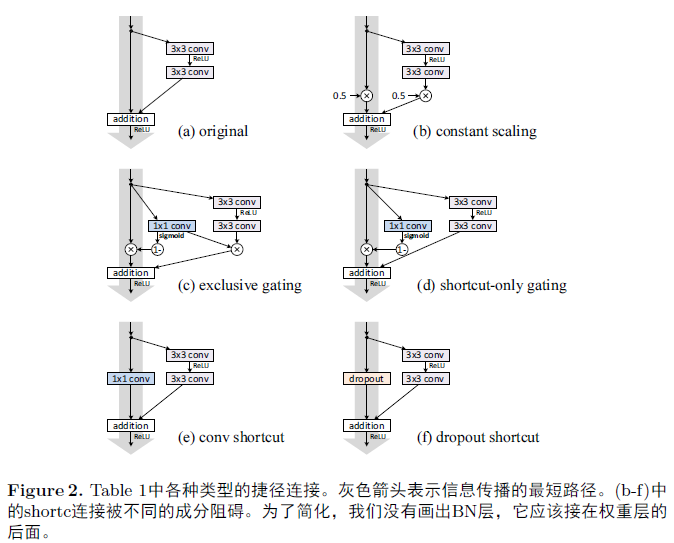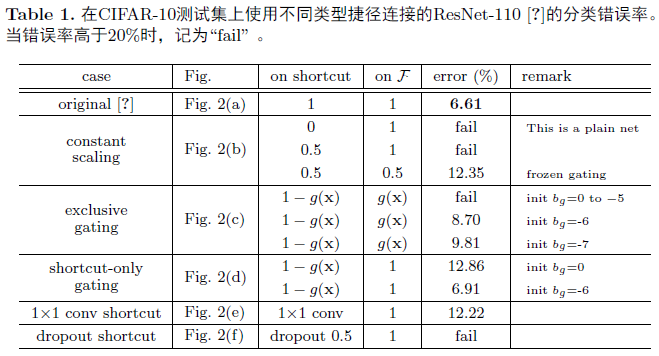3.1 Experiments on Skip Connections

Constant scaling 对于所有的捷径连接，我们设置λ=0.5$\lambda=0.5$(Fig.2(b))。我们进一步考虑F$\mathcal{F}$ 的两种缩放情况： (i) F$\mathcal{F}$不被缩放; (ii) F$\mathcal{F}$ 有常数标量 1λ=0.5$1-\lambda=0.5$进行缩放，这与highway门控类似，但是它是冷冻门控。前一种情况不能很好的收敛；后一种可以收敛，但是测试错误率(Table1, 12.35%)比原始的ResNet-110高得多。Fig.3(a) 显示了训练错误率比原始ResNet-110的要高，表明了当捷径信号被缩放时，优化变得更加困难。

Exclusive gating 根据采用门控机制的Highway Networks，我们设计一个门控函数g(x)=σ(Wgx+bg)$g({x})=\sigma({W}_g{x}+b_g)$ ，表示由权重Wg${W}_g$ 、偏置项bg$b_g$以及sigmoid函数σ(x)=11+ex$\sigma(x)=\frac{1}{1+e^{-x}}$组成的变换。在一个卷积网络中g(x)$g({x})$ 通过 1×1$\times1$的卷积层实现。门控函数通过元素乘法来调节信号。

Exclusive gating机制的影响是双面的。当 1g(x)$1-g({x})$ 接近1时，门控捷径连接接近于恒等映射，这有助于信息的传递；但是这种情况下，g(x)$g({x})$ 接近于0，从而阻碍了函数F$\mathcal{F}$。 为了避免在捷径连接中门控函数的影响，我们在接下来研究一个non-exclusive的门控机制。

Shortcut-only gating 这种情况下，函数F$\mathcal{F}$不进行缩放；只有捷径连接由1g(x)$1-g({x})$进行缩放。如Fig.2(d)所示。偏置项bg$b_g$的初始值同样很重要。当bg$b_g$的初始值为0时(即1g(x)$1-g({x})$ 为 0.5)，网络收敛到一个很差的结果 12.86% (Table1)。这同样产生了很高的训练错误率(Fig.3(c))。

bg$b_g$的初始值是负很多的数(例如, 6$-6$)时，1g(x)$1-g({x})$的值接近于1，捷径连接接近于恒等映射。因此，结果(6.91%, Table1)与原始ResNet-110的结果很接近。

1×$\times$1 convolutional shortcut 接下来我们使用 1×$\times$1 的卷积捷径连接替代恒等连接在进行实验。这种方案在He2016中使用在34层的ResNet(16个残差单元)上(即方案C)，并展现除了很好的结果，表明了1×$\times$1 卷积捷径连接是有效果的。但是我们发现当残差单元有很多时，这并不能起到特别好的效果。当使用1×$\times$1 的卷积捷径连接时，110 层的ResNet的结果很差(12.22%, Table1)。同样的训练误差也变得很高 (Fig.3(d))。当堆叠了如此多的残差单元时(ResNet-110 中有54个)，即便是最短的路径可能也会对信号的传播造成阻碍。当在ImageNet上使用1×$\times$1卷积捷径连接的ResNet-110时，出现了类似的结果。

Dropout shortcut 最后我们在恒等捷径连接上添加Dropout(比率为0.5) 来进行实验(Fig.2(f))。网络并没有收敛到一个很好的结果。Dropout在统计学上相当于给捷径连接强加了一个λ$\lambda$为0.5的缩放，这和0.5的constant scaling很类似，同样的阻碍了信号的传播。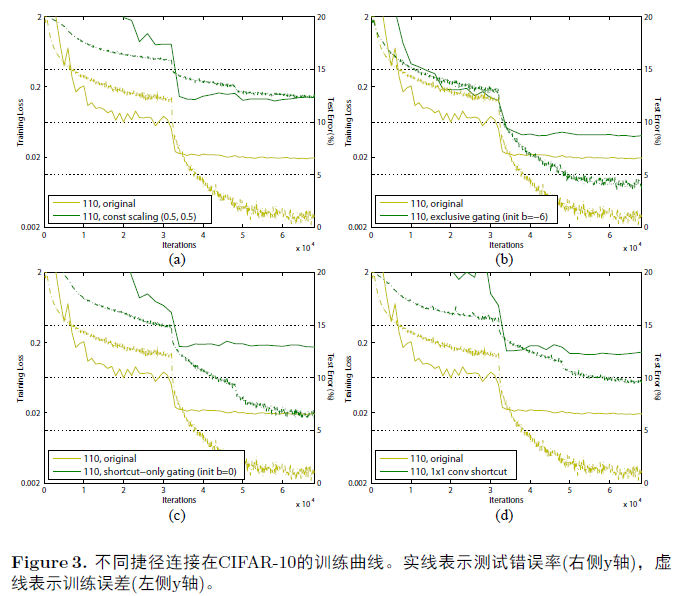3.2 Discussions

## On the Usage of Activation Functions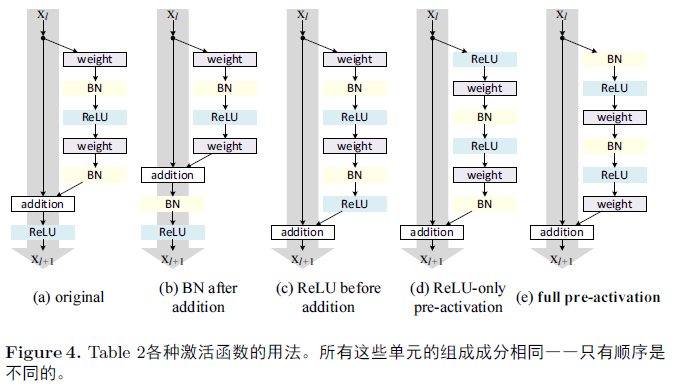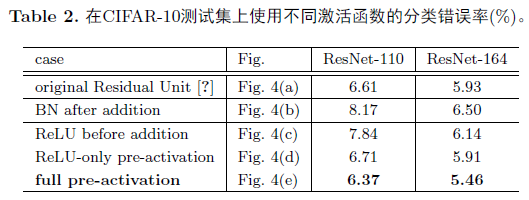4.1 Experiments on Activation

BN after addition 在将f$f$调整至恒等映射之前，我们先反其道而行之，在加法后添加一个BN(Fig.4(b))。这样f$f$ 就包含了BN和ReLU。这样的结果比基本结构的结果要差很多(Table2)。不像原始的设计，目前的BN层改变了流经捷径连接的信号，并阻碍了信息的传递，这从训练一开始降低训练误差的困难就可以看出(Fig.6 left)。

ReLU before addition 使得f$f$成为恒等映射的一个天真的选择就是将 ReLU 移到加法之前(Fig.4(c))。这导致了 F$\mathcal{F}$的输出为非负，然而一个“残差”函数的输出应该是(,+)$(-\infty, +\infty)$ 的。造成的结果就是，前向传递的信号是单调递增的。这会影响表达能力，结果也变得更差了(7.84%, Table2) 。我们希望残差函数的值是在区间(,+)$(-\infty, +\infty)$中的。这个条件可以由包括以下的残差单元来实现。

Post-activation or pre-activation?

xl+1=xl+F(f^(xl),Wl),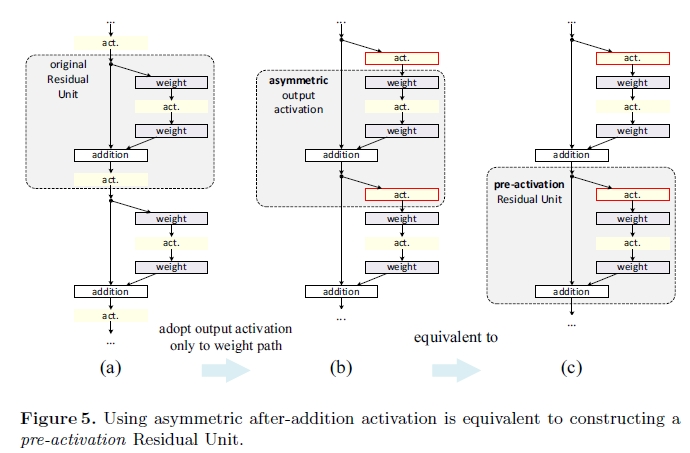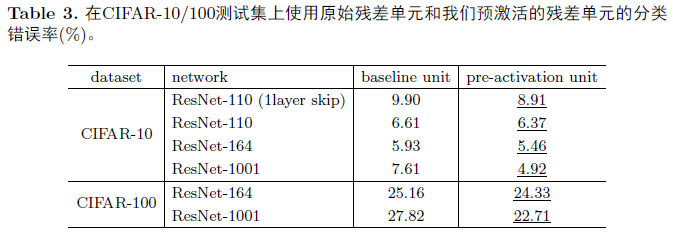4.2 Analysis

Ease of optimization 这个影响在我们训练1001层ResNet时尤为显著。Fig.1展示了训练和测试曲线。使用He2016中的原始结构时，训练错误率在一开始下降的特别慢。对于f=$f=$ ReLU，如果信号是负的时候会造成一定的影响，而当残差单元很多时，这个影响将会变得尤为突出，所以Eq.3(Eq.5也是)并不是一个好的估计。另一方面，当f$f$时一个恒等映射时，信号在两个单元之间能够很直接的进行传递。我们的1001层网络的训练误差能够降低的特别快(Fig.1)。它同样达到了所有我们研究的模型中最低的误差，表明了在优化上的成功。

Reducing overfitting 使用这个预激活单元的另一个影响就是正则化，如Fig.6(右)所示。预激活版本的模型达到收敛时产生了稍高的训练损失，但是却只产生了最低的的测试错误率。这个现象在CIFAR-10和100上的ResNet-110，ResNet-110(1-layer)以及ResNet-164上都观测到了。这可能是由BN的正则化效应引起的。在原始残差单元中(Fig.4(a))，尽管BN对信号进行了标准化，但是它很快就被合并到捷径连接上，组合的信号并不是被标准化的。这个非标准化的信号又被用作下一个权重层的输入。恰恰相反的是，在我们的预激活版本的模型中，权重层的输入总是标准化的。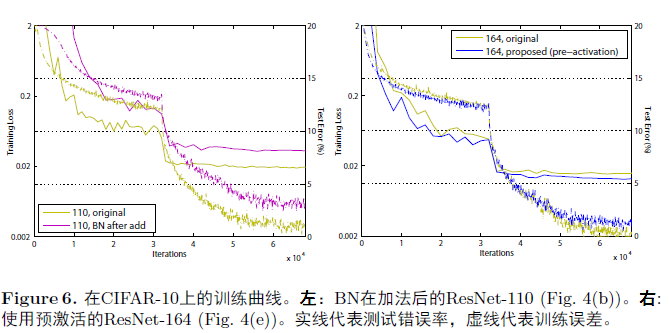## Results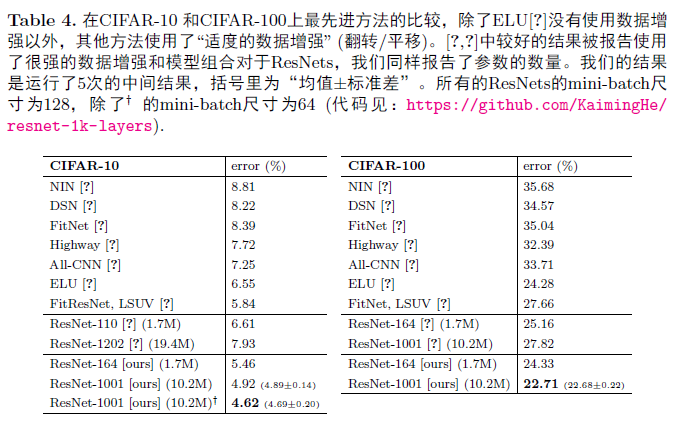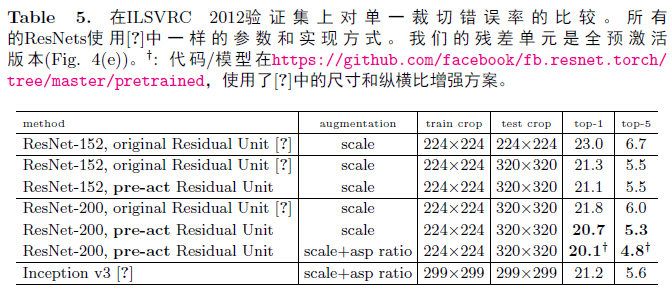Comparisons on CIFAR-10/100
Table4在 CIFAR-10/100上比较了目前最好的方法，我们的模型取得了非常有竞争性的结果。我们发现对于这些小数据集，我们并不需要特意剪裁网络的宽度和深度，也不需要使用正则化技术(例如dropout)来保证模型的效果。我们只通过一个简单却有效的方式——加深网络。这些结果展示了推进深度极限的潜力。

Comparisons on ImageNet

Table5展示了ResNet-152和 ResNet-200的结果，所有训练从头开始。我们注意到原始的ResNet论文中在训练模型时，使用了图像较短边s[256,480]$s\in[256, 480]$的尺寸抖动(scale jittering)，所以当s=256$s=256$时，在224×$\times$224的裁切图像的测试上加了负的偏置。相反的，在所有原始的以及我们提出的ResNet上，我们从s=320$s=320$的图像中裁切一个单一的320×$\times$320图像进行测试。

Computational Cost

## Appendix: Implementation Details

11-184289

#### 论文笔记（一）. Identity mapping in Deep Residual Networks07-043704

#### 论文笔记 | Identity Mappings in Deep Residual Networks

06-29813

#### 综述论文翻译：A Review on Deep Learning Techniques Applied to Semantic Segmentation

12-082万+

#### 深度残差网络（Deep Residual Network ）

02-20102

#### 翻译 - ResNet-v2

02-032567

#### Pytorch实现ResNet V2-Pre-activation ResNet

01-212万+

#### 深度学习——残差神经网络ResNet在分别在Keras和tensorflow框架下的应用案例©️2020 CSDN 皮肤主题: 大白 设计师: CSDN官方博客点击重新获取扫码支付1.余额是钱包充值的虚拟货币，按照1:1的比例进行支付金额的抵扣。
2.余额无法直接购买下载，可以购买VIP、C币套餐、付费专栏及课程。余额充值